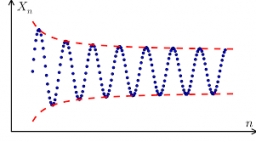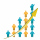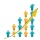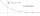# Missing term 2

What is the missing term for the Geometric Progression (GP) 3, 15, 75,__, 1875?

a4 =  375

### Step-by-step explanation:Did you find an error or inaccuracy? Feel free to write us. Thank you!## Related math problems and questions:

• Arithmetic progression 2The 3rd term of an Arithmetic progression is ten more than the first term, while the fifth term is 15 more than the second term. Find the sum of the 8th and 15th terms of the Arithmetic progression if the 7th term is seven times the first term.
• Sum 1-6Find the sum of the geometric progression 3, 15, 75,… to six terms.
• What are 2What are the two more terms of the GP a, ax, ax2, ax3, __, __?
• 15th term of APWhat is the 15th term; x1=1.5, d=4.5?
• What is 10What is the 5th term, if the 8th term is 80 and common ratio r =1/2?
• Missing termWhat is the missing term in the sequence? _____, 13, 30, and 47?
• GP - three membersThe second and third of a geometric progression are 24 and 12(c+1), respectively, given that the sum of the first three terms of progression is 76. determine the value of c.
• Terms of GPWhat is the 6th term of the GP 9, 81, 729,. .. ?
• SequenceCalculate what member of the sequence specified by (5n-2+15) has value 20.
• Three members GPThe sum of three numbers in GP (geometric progression) is 21 and the sum of their squares is 189. Find the numbers.
• Five membersWrite first 5 members geometric sequence and determine whether it is increasing or decreasing: a1 = 3 q = -2
• SequenceFind the common ratio of the sequence -3, -1.5, -0.75, -0.375, -0.1875. Ratio write as decimal number rounded to tenth.
• Two geometric progressionsInsert several numbers between numbers 6 and 384 so that they form with the given GP numbers and that the following applies: a) the sum of all numbers is 510 And for another GP to apply: b) the sum of entered numbers is -132 (These are two different geome
• Elimination methodSolve system of linear equations by elimination method: 5/2x + 3/5y= 4/15 1/2x + 2/5y= 2/15
• Population growthHow many people will be on Earth from two people for 5,000 years, if every couple has always 4 children, (2 boys and 2 girls) at the age of 25-35, and every man will live 75 years?
• If the 3If the 6th term of a GP is 4 and the 10th is 4/81, find common ratio r.
• Sum of GP membersDetermine the sum of the GP 30, 6, 1.2, to 5 terms. What is the sum of all terms (to infinity)?